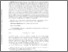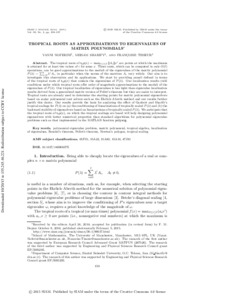# Tropical roots as approximations to eigenvalues of matrix polynomials

Noferini, V and Sharify, M and Tisseur, F (2015) 'Tropical roots as approximations to eigenvalues of matrix polynomials.' SIAM Journal on Matrix Analysis and Applications, 36 (1). 138 - 157. ISSN 0895-4798Preview
Text
14096637x.pdf - Published Version
Available under License Creative Commons Attribution.

Download (412kB) | Preview

## Abstract

The tropical roots of t p(x) = max ∥A ∥x are points at which the maximum is attained for at least two values of i for some x. These roots, which can be computed in only O (ℓ) operations, can be good approximations to the moduli of the eigenvalues of the matrix polynomial P (λ) = Σ λ A , in particular when the norms of the matrices A vary widely. Our aim is to investigate this observation and its applications. We start by providing annuli defined in terms of the tropical roots of t p (x) that contain the eigenvalues of P (λ). Our localization results yield conditions under which tropical roots offer order of magnitude approximations to the moduli of the eigenvalues of P (λ). Our tropical localization of eigenvalues is less tight than eigenvalue localization results derived from a generalized matrix version of Pellet's theorem but they are easier to interpret. Tropical roots are already used to determine the starting points for matrix polynomial eigensolvers based on scalar polynomial root solvers such as the Ehrlich-Aberth method and our results further justify this choice. Our results provide the basis for analyzing the effect of Gaubert and Sharify's tropical scalings for P (λ) on (a) the conditioning of linearizations of tropically scaled P (λ) and (b) the backward stability of eigensolvers based on linearizations of tropically scaled P (λ). We anticipate that the tropical roots of t p(x), on which the tropical scalings are based will help designing polynomial eigensolvers with better numerical properties than standard algorithms for polynomial eigenvalue problems such as that implemented in the MATLAB function polyeig. x 0≤ i≤ℓ i i=0 i i x x i ℓ i

Item Type: Article Q Science > QA Mathematics Faculty of Science and Health > Mathematical Sciences, Department of Jim Jamieson 20 Oct 2015 13:38 31 Mar 2021 07:15 http://repository.essex.ac.uk/id/eprint/15324

### Actions (login required)View Item